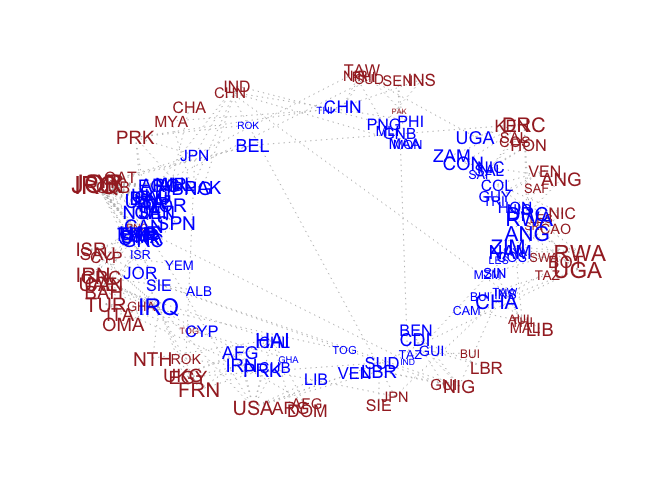## amen

#### Additive and multiplicative effects network models

Package websiteAdditive and multiplicative effects network models (AMEN models) provide a statistical modeling framework for dyadic network and relational data, built upon familiar data analysis tools such as linear regression, random effects models and matrix decompositions. The amen package provides Bayesian model fitting algorithms for AMEN models, and accommodates a variety of types of network relations, including continuous, binary and ordinal dyadic variables.

The basic AMEN model is of the form yi, j ∼ βxi, j + uivj + ai + bj + ϵi, j where

• yi, j is the observed dyadic variable being modeled and xi, j is an observed vector of regressors;

• ai + bj + ϵi, j is an additive random effects term that describes sender and receiver variance (such as outdegree and indegree heterogeneity) and dyadic correlation;

• uivj is a multiplicative random effects term that describes third-order dependence patterns (such as transitivity and clustering) and can be estimated and analyzed to uncover low-dimensional structure in the network.

#### Installation

# Current version on GitHub
devtools::install_github("pdhoff/amen")

# CRAN-approved version on CRAN
install.packages("amen")

#### Documentation and citation

A tutorial article and many data analysis examples are available via the tutorial. Please cite this as

Hoff, P.D. (2015) “Dyadic data analysis with amen”. arXiv:1506.08237.

A review article that provides some mathematical details and derivations is available on arXiv. Please cite this

Hoff, P.D. (2018) “Additive and multiplicative effects network models”. arXiv:1807.08038.

The first version of the AMEN model appeared in

Hoff, P.D. (2005) “Bilinear mixed-effects models for dyadic data”. JASA 100(469) 286-295.

That version restricted the multiplicative sender and receiver effects to be equal (ui = vi). The AMEN model in its current form does not have this restriction. The current AMEN model first appeared in

Hoff, P.D., Fosdick, B.K., Volfovsky, A. and Stovel, K. (2013) “Likelihoods for fixed rank nomination networks”. Network Science, 1(3):253–277.